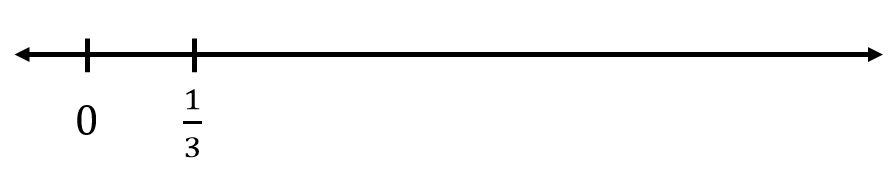# Identify a Fraction on a Number Line

Directions: Label the point where 3/4 belongs on the number line. Be as precise as possible.### Hint

How can we figure out where one goes? How can we use that information to figure out where 3/4 goes?

Source: Inspired by Illustrative Mathematics

## Make The Time

Directions: Using the digits 0 to 9 at most one time each, place a digit …

1.between 2/4 and 4/4

••venom is cool im a girl

ok

•Thanks guys 😘

2.between 2/4 and 4/4

3.Between 2/4 and one whole

4.John Birnstengel

I agree with Orson and Clive.

5.3/4 on the number is between 2/4 and 4/4.

6.By agree I mean I agree with Clive

7.I think it is between 2/4 and 4/4

8.In the middle of 2/4 and 1.

9.In the middle of 2/4 and a whole

10.11.3/4 is on the number between 4/4 and 2/4.

••You will never know

I agree

12.I think that it is in the middle of 2/4 and 4/4

13.14.between 2/4 and 4/4

15.I guess we answer here??? between 2/4 and 4/4. I guess we use mom’s email address? I’m 8. I don’t have email. What is this??

16.between 2/4 and 4/4.I don’t have an email so I will just use my moms.

17.Between 2/4 and 4/4 it is 3/4.

18.between 2/4 and 4/4

19.inbetween 2/3 and 3/3

20.probly in between 2/3 and and 3/3

21.Everybody knows its in between 2/4 and 4/4 AKA 1.

22.I agree with kayla

23.Scarlett Salaz

Between 1/2 and 1/1

24.3/4 is between 2/4 and 4/4

25.I wonder if it’s possible to open this question even further, by leaving the 0, and asking students to place both the 1/3 and the 3/4? Or remove the 0, and then label 0, 1, and 3/4. Might provide more interesting solutions for discussions afterwards?

26.the middle of 1 whole and 2/4

27.In-between 2/4 and 4/4 but still not exactly in the middle

28.I think between 2/4 and 4/4

29.In between 2/4 and 4/4

30.It would be 1/4 away from the whole and there would be 4 spaces

31.In between 2/4 and 4/4 or 1